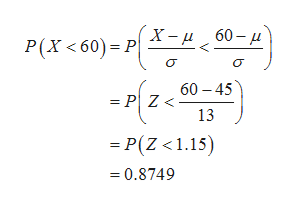# Suppose the mean income of firms in the industry for a year is 4545 million dollars with a standard deviation of 13 million dollars. If incomes for the industry are distributed normally, what is the probability that a randomly selected firm will earn less than 60 million dollars? Round your answer to four decimal places.

Question
4 views

Suppose the mean income of firms in the industry for a year is 4545 million dollars with a standard deviation of 13 million dollars. If incomes for the industry are distributed normally, what is the probability that a randomly selected firm will earn less than 60 million dollars? Round your answer to four decimal places.

check_circle

Step 1

Given information:

Step 2

Let X is random variable.

The probability that a randomly selected firm wi...help_outlineImage TranscriptioncloseX- u 60 - u P(X <60)= P --{2-) 60 – 45 = P| Z < 13 = P(Z <1.15) = 0.8749 fullscreen

### Want to see the full answer?

See Solution

#### Want to see this answer and more?

Solutions are written by subject experts who are available 24/7. Questions are typically answered within 1 hour.*

See Solution
*Response times may vary by subject and question.
Tagged in

### Measures